# descriptive geometry

Also found in: Dictionary, Thesaurus, Wikipedia.

## descriptive geometry,

branch of geometrygeometry
[Gr.,=earth measuring], branch of mathematics concerned with the properties of and relationships between points, lines, planes, and figures and with generalizations of these concepts.
concerned with the two-dimensional representation of three-dimensional objects; it was introduced in 1795 by Gaspard Monge. By means of such representations, geometrical problems in three dimensions may be solved in the plane. (Such problems arise in all branches of engineering.) Modern mechanical drawing and architectural drawing are based on the principles of descriptive geometry.

## Descriptive Geometry

a branch of geometry in which we study three-dimensional objects by investigating their planar representations, in particular, their projections. Descriptive geometry also studies methods of solving spatial problems on a plane.

The representation of three-dimensional objects on a plane was necessitated by various practical problems that arose during the construction of buildings and other structures and in the development of painting, architecture, and technology. Mechanical drawings obtained by projecting figures on a plane (projective drawings) are of particular importance. Actual practice imposes a number of requirements on such drawings. The most important are (1) ease of visualization of the original object; (2) “invertibility” of the drawing, that is, the possibility of exactly determining the depicted object from the drawing; (3) simplicity of carrying out the required constructions; and (4) accuracy of the graphical solutions.

Central and parallel projections are used to represent three-dimensional objects. It is easiest to visualize objects on the basis of drawings obtained by central projection. (The geometry of central projection resembles the geometry of formation of images on the retina of the human eye.) However, widest use is made of various types of parallel projection. Parallel projections are easy to construct, and it is also relatively easy to reconstruct from them the original figures. The most popular type of parallel projection is orthogonal, or orthographic, projection on two or three planes (composite drawing). In this method, we select in space two mutually perpendicular projection planes Π1 and Π2 referred to as the horizontal and vertical plane of projection, respectively. An arbitrary point A in space is orthogonally projected in these planes (Figure 1), yielding the horizontal projection A1 (AA1 ⊥ 1 Π1) and the vertical projection A2 (AA2 ⊦ Π2).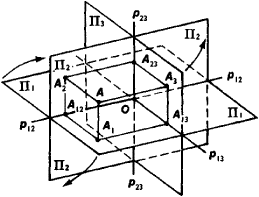Figure 1

The three points A, A1, and A2 lie in the same (projecting) plane perpendicular to the intersection line ρ2 of the projection planes. The horizontal and vertical projections of an object are obtained by orthogonally projecting all the points of the object on Π 1 and Π 2, respectively. It is often useful to add a third projection of the object on a plane Π 3 perpendicular to Π1 and Π 2. The plane Π 3 is called the profile plane, and the projection on it is called the profile. Two projections of A (for example, A1 and A2) completely determine the third (A3).

To obtain a drawing consisting of the three projections (composite drawing), we superimpose Π1` and Π 3 on Π 2 (principal plane) by rotation about the lines p12 and P23 in which these planes intersect Π 2 (Figure 2). The positions of the lines P12 and P23 are usually not indicated in practice, that is, the positions of the projection planes are determined to within parallel displacement translations.Figure 2

A composite drawing is invertible since it is possible to determine from it the distance between any two points of the actual object. In fact, the segment AB (Figure 3) is the hypotenuse of the right triangle ABB*, in which AB* = A1B1, while B*B is the difference in the altitudes of B and A, represented in the drawing by the segment B2*B B2. It is thus possible to obtain the actual segment AB = A1 (Figure 4) by jneans of a simple construction: all we need to do is draw B1B̄ ⊦ A1B1 and B1B̄ = B2 * B2. To make it easier to visualize an object on the basis of a composite drawing we add “visibility conditions” to the projections: of two points A and B whose projections on one of the projection planes coincide (for example, A1 ≡ B1), the visible point is the point nearest the observer, and hidden lines are represented by dashed lines. Figure 5 shows such a representation of a three-dimensional object by means of three projections called, respectively, the front view (elevation), top view (plan), and end view (profile).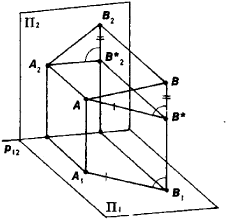Figure 3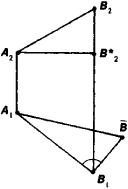Figure 4

A composite drawing (made up of two or three orthogonal projections) is the most frequently used type of engineering drawing. All the necessary dimensions of a depicted object are easily determined from such a drawing. What it lacks is ease of visualization of the object. To obtain representations that make visualization easier we use a method called axonometry.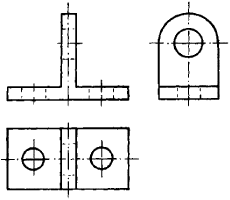Figure 5

In axonometry, an object is represented in a coordinate system Oxyz in space. This coordinate system is called the natural system. Figure 6 shows a coordinate broken line OMxM1M for an arbitrary point M. The lengths of the coordinate segments OMx, MX M1, and M1M are the coordinates x, y, and z of M. If we project a natural coordinate system Oxyz on a plane Π’, then we obtain a so-called axonometric system of axes O’ x’ y’ z’ (Figure 6). The projection O’ MxMiM’ of the coordinate broken line consists of the segments O’ M’ x, M’ xMi, and MiM’ whose lengths x’, y’, and z’ in the axonometric coordinate systems are called the axonometric coordinates of M. The ratios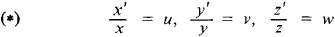express the distortion of the coordinate segments under projection and are called the distortion coefficients. The axonometry is called isometric if the three distortion ratios are equal, dimetric if at least two of the ratios are equal, and trimetric if they are all unequal.

In order to construct on a plane Π’ the image of a point MFigure 6

under a given parallel projection using the axonometric method, we must have the natural coordinates of the point M(x, y, z), an axonometric axis system O’x’y’z’ on the projection plane II, and the distortion ratios u, v, w. The axonometric coordinates of the point M’(x’, y’, z’) are then found from the formulas (*) and are used to construct the projection M’ of M. The totality of projections M’ yields the axonometric representation of the three-dimensional object. An axonometric drawing is invertible: Given a point M’(x’, y’, z’) of an axonometric drawing, it is possible to find the natural coordinates x, y, and z of M by means of the formulas (*).

The following assertion (Polke’s theorem) is known as the fundamental theorem of axonometry. For a given axonometric axis system O’x’y’z’ (not a line) on the projection plane IT and a given ratio u:v:w of the distortion coefficients, we can find a position of the natural coordinate system relative to Π’ and a direction of projection that realize the selected axonometric axis system in IT and the ratios of the distortion coefficients.

To simplify the axonometric method we use a “reduced” axonometry, in which the axonometric coordinates are replaced inasmuch as possible by the natural coordinates without distortion of the form of the drawing. Figure 7 represents an orthogonal isometric view of the object depicted in the composite drawing in Figure 5 using natural instead of axonometric coordinates. In this case, the scale of the axonometric drawing changes, but the appearance is preserved, that is, the drawing changes homothetically. Objects that are not greatly elongated can be easily visualized using their axonometric representations. This cannot be said of representations of large objects, such as buildings and dams. In these cases, it is preferable to use representations obtained by central projection (perspective representations).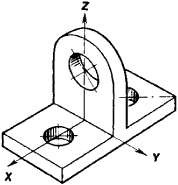Figure 7

To make a perspective (drawing) invertible it is necessary to construct on the projection plane Π’ the central projection A’ (perspective) of the depicted point A and the perspective A1’ of the orthogonal projection A1 of the point on the horizontal plane Π1, called the ground plane (Figure 8). The plane of projection Π’ (picture plane) is preferably selected perpendicular to the ground plane. A1 is then called the foot of the point A. In particular, S1 is the foot of the station point (“eye”) 5. If we know the position of S relative to II, then we can find the position of the point A in space using as data the perspective A’ of A and the perspective A1 of its foot. To this end we draw SA’1 and find A1. We then construct A1A 1 Π1 and find the point A at the intersection of the lines SA’ and A1A. Vanishing points, which are perspective representations of infinitely distant points in space, and the horizon line, which is the perspective representation of the infinitely distant line of the ground plane Π1, are of great importance in perspective constructions.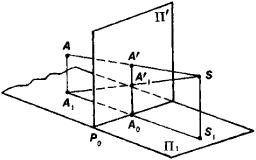Figure 8

A perspective of a room is depicted in Figure 9. The figure shows both the principal point y’, which is the vanishing point for all lines perpendicular (in reality) to the picture plane, and the horizon line h. The vanishing points of other parallel lines lying in the ground plane are on the horizon line h (for example, D’Figure 9

Using coordinates it is possible to construct a perspective employing central instead of parallel axonometry. In addition to perspective constructions on the plane (linear perspective), other types of central projection representations are also used.

Projections with number marks are used in preparing relief maps of the earth. The process is suggested in Figure 10. In this case, we must start with a sufficient number of points of the depicted surface in the drawing (Figure 10). Points of the surface are orthogonally projected onto the plane of projection, and the elevation of every point, that is, a number expressing the height of a point above the plane of projection in the selected unit of length, is written near the projection. As a result, the drawing is invertible. Projections of points with identical heights are connected by lines, called contour, or level, lines. This increases the clarity of the drawing and facilitates its use. In depicting the earth’s surface, we assume the plane of projection to be horizontal, and the contour lines are called horizontals. The shape and disposition of the horizontals enable us to determine the relief of the depicted part of the earth’s surface, to construct the surface’s cross-section specified by a plane σ on the drawing (Figure 10), and to solve other problems. Accuracy depends on the initial data. This method of depicting a surface is called the topographic method, and maps obtained by this method are called topographic.

History. The first attempts to create projective representations were made by man long before the Common Era. The Roman architect Vitruvius in his De architectura (first century B.C.) introduced the concepts of a plan (horizontal projection) and facade (front projection) of buildings. The Italian architect and scientist L. Alberti (15th century) already made use of vanishing points and provided a method of practical importance for constructing perspectives by means of networks. Leonardo da Vinci’s Treatise on Painting (published 1651) contains numerous suggestions for practical applications of perspective representations and, in particular, “observational” perspective. The German artist A. Diirer, in his Course in the Art of Measurement (1525), proposed a method of constructing perspectives using horizontal and vertical projections of an object. A rather complete presentation of methods of constructing perspectives was given by the Italian scientist G. Ubaldo (1600). The scientific foundations of descriptive geometry were developed in part by G. Desargues; however, it is G. Monge who is considered the founder of scientific descriptive geometry and contributed most to the subject.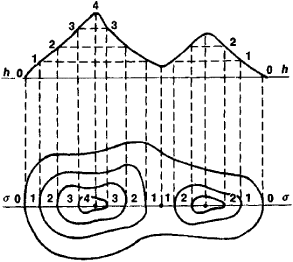Figure 10

In ancient Russia drawings with elements of geometric projection were used in the construction of buildings. There exists a drawing (1581) of the city of Pskov using several laws of perspective. The drawings of the self-educated inventor I. P. Kulibin, the architect D. V. Ukhtomskii, and others are geometrically correct projective images. A course in descriptive geometry was first introduced in 1810 at the St. Petersburg Institute of Railroad Engineers. Ia. A. Sevast’ianov, the first Russian professor of descriptive geometry, wrote a number of texts dealing with various problems in descriptive geometry. The scientific development of descriptive geometry was given impetus by the geometric studies of E. S. Fedorov, who proposed a method of representing points in space on a plane by means of vectors. Fedorov’s method has been effectively applied to multidimensional descriptive geometry, which is used in physicochemical analysis (N. S. Kurnakov school). Soviet geometers (A. K. Vlasov, N. A. Glagolev, N. F. Chetverukhin) have carried out a number of investigations on the fundamental theorem of axonometry.

### REFERENCES

Rynin, N. A. Materialy k istorii nachertatel’noi geometrii: Bibliografiia, biografii, epizody, fakty, khronologiia. Leningrad, 1938.
Monge, G. Nachertatel’naia geometriia. Moscow, 1947. (Translated from French.)
Fedorov, E. S. “Novaia nachertatel’naia geometriia.” Izv. AN, 1917, no. 10.
Glagolev, N. A. Nachertatel’naia geometriia, 3rd ed. Moscow, 1953.
Vol’berg, O. A. Lektsiipo nachertatel’noi geometrii. Moscow-Leningrad, 1947.
Kurs nachertatel’noi geometrii. Edited by N. F. Chetverukhin. Moscow, 1956.
Voprosy sovremennoi nachertatel’noi geometrii Collection of articles edited by N. F. Chetverukhin. Moscow-Leningrad, 1947.
Glazunov, E. A. , and N. F. Chetverukhin. Aksonometriia. Moscow, 1953.
Metody nachertatel’noi geometrii i ee prilozheniia. Collection of articles edited by N. F. Chetverukhin. Moscow, 1955.
Dobriakov, A. I. Kurs nachertatel’noi geometrii, 3rd ed. Moscow-Leningrad, 1957.

N. F. CHETVERUKHIN

## descriptive geometry

[di′skrip·tiv jē′äm·ə·trē]
(mathematics)
The application of graphical methods to the solution of three-dimensional space problems.
References in periodicals archive ?
These EVEs should have tools that measure student learning and Web3D models as a resource to support learning and to improve spatial visualization skills, formerly acquired in descriptive geometry classes.
The graphic expression study module at our university is divided into four parts: technical drawing, geometry, descriptive geometry and CAD.
The traditional way of teaching descriptive geometry and engineering graphics with pencil, T-square and triangles will be more and more replaced by electronic data processing.
ASPECTS OF CAD TECHNIQUES USED IN THE DESCRIPTIVE GEOMETRY STUDY
Using the CAD techniques implies the assimilation by students not only of the descriptive geometry but also of the methods for geometrically modeling and defining CAD features.
Nevertheless, teaching the descriptive geometry should take into consideration its essential specific purposes: improving visually geometric reasoning, training the spatial view and ability to transfer information between 2D and 3D space mutually.
Under the above mention considerations, the study of a number of bibliographical books and papers in the field, the study plans in different worldwide engineering universities and their own attempts, the authors consider as necessary the assimilation of the 3D-CAD modeling techniques before learning the descriptive geometry advanced ideas (solids representations, solids and evolutes intersections).
Under these conditions, the descriptive geometry study should consist in two training stages; the first including essential elements and the second the advanced elements.
In order to acquire efficiency in teaching the descriptive geometry, the CAD techniques should be implemented according to a strict plan based on a unitary discipline outlook and a structure, with clearly defining the purpose and modeling methods used, and creating some best working algorithms.
Teacher should define these elements under the specific conditions of the descriptive geometry.
The classic method of training in the descriptive geometry field has very good results in their case.
THE ADVANTAGES OF USING COMPUTER FOR THE DESCRIPTIVE GEOMETRY

Site: Follow: Share:
Open / Close